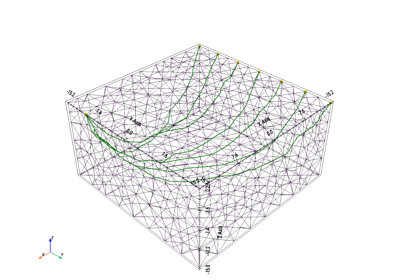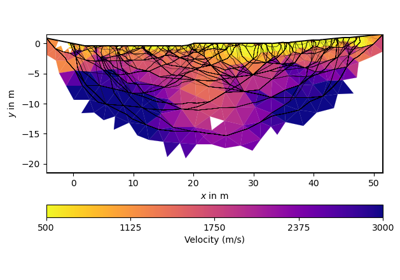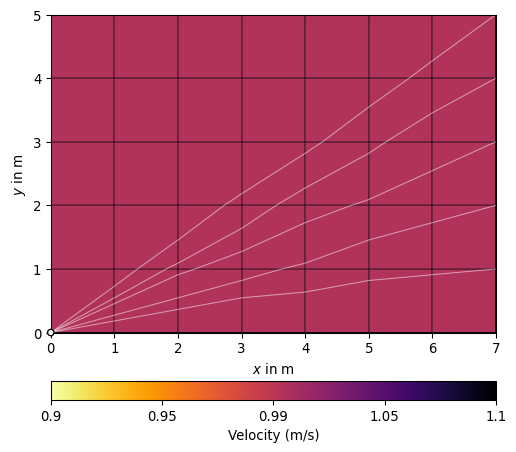# pygimli.physics.traveltime¶

Refraction seismics or first arrival traveltime calculations.

## Overview¶

Functions

 createGradientModel2D(data, mesh, vTop, vBot) Create 2D velocity gradient model. createRAData(sensors[, shotDistance]) Create a refraction data container. drawFirstPicks(ax, data[, tt, plotva, marker]) plot first arrivals as lines drawTravelTimeData(ax, data[, t]) Draw first arrival traveltime data into mpl ax a. drawVA(ax, data[, vals, usePos, pseudosection]) Draw apparent velocities as matrix into ax load(fileName[, verbose]) Shortcut to load TravelTime data. shotReceiverDistances(data[, full]) Return vector of all distances (in m) between shot and receiver.

Classes

 RefractionNLayer([offset, nlay, vbase, verbose]) Forward operator for 1D Refraction seismic with layered model. RefractionNLayerFix1stLayer([offset, nlay, …]) FOP for 1D Refraction seismic with layered model (e.g. TravelTimeDijkstraModelling(**kwargs) Forward modelling class for traveltime using Dijsktras method. TravelTimeManager([data]) Manager for refraction seismics (traveltime tomography).

## Functions¶

pygimli.physics.traveltime.createGradientModel2D(data, mesh, vTop, vBot)[source]

Creates a smooth, linear, starting model that takes the slope of the topography into account. This is done by fitting a straight line and using the distance to that as the depth value. Known as “The Marcus method”

Parameters
• data (pygimli DataContainer) – The topography list is in here.

• mesh (pygimli.Mesh) – The parametric mesh used for the inversion

• vTop (float) – The velocity at the surface of the mesh

• vBot (float) – The velocity at the bottom of the mesh

Returns

model – A numpy array with slowness values that can be used to start the inversion.

Return type

pygimli Vector, length M

pygimli.physics.traveltime.createRAData(sensors, shotDistance=1)[source]

Create a refraction data container.

Default data container for shot and geophon at every sensor position. Predefined sensor indices’s ‘s’ as shot position and ‘g’ as geophon position.

Parameters
• sensors (ndarray | R3Vector) – Geophon and shot positions (same)

• shotDistances (int ) – Distance between shot indieces.

Returns

data – Data container with predefined sensor indieces ‘s’ and ‘g’ for

Return type

DataContainer

Examples using pygimli.physics.traveltime.createRAData### drawFirstPicks¶

pygimli.physics.traveltime.drawFirstPicks(ax, data, tt=None, plotva=False, marker='x-')[source]

plot first arrivals as lines

Examples using pygimli.physics.traveltime.drawFirstPicks### drawTravelTimeData¶

pygimli.physics.traveltime.drawTravelTimeData(ax, data, t=None)[source]

Draw first arrival traveltime data into mpl ax a. data of type

ef DataContainer must contain sensorIdx ‘s’ and ‘g’

and thus being numbered internally [0..n)

### drawVA¶

pygimli.physics.traveltime.drawVA(ax, data, vals=None, usePos=True, pseudosection=False, **kwargs)[source]

Draw apparent velocities as matrix into ax

Parameters
• ax (mpl.Axes) –

• data (pg.DataContainer()) – Datacontainer with ‘s’ and ‘g’ Sensorindieces and ‘t’ traveltimes.

• usePos (bool [True]) – Use sensor positions for axes tick labels

• pseudosection (bool [False]) – Show in pseudosection style.

• vals (iterable) – Traveltimes, if None data need to contain ‘t’ values.

pygimli.physics.traveltime.load(fileName, verbose=False, **kwargs)[source]

Import Data and try to assume the file format.

TODO

Parameters

fileName (str) –

Returns

data

Return type

pg.DataContainer

pygimli.physics.traveltime.shotReceiverDistances(data, full=False)[source]

Return vector of all distances (in m) between shot and receiver. for earch ‘s’ and ‘g’ in data.

Parameters
• data (pg.DataContainerERT) –

• full (bool [False]) – Get distances between shot and receiver posisiton when full is True or only form x coordinate if full is False

Returns

dists – Array of distances

Return type

array

## Classes¶

RefractionNLayer

class pygimli.physics.traveltime.RefractionNLayer(offset=0, nlay=3, vbase=1100, verbose=True)[source]

Bases: pygimli.core.ModellingBaseMT__

Forward operator for 1D Refraction seismic with layered model.

__init__(offset=0, nlay=3, vbase=1100, verbose=True)[source]

Init forward operator. Model are velocity increases.

Parameters
• offset (iterable) – vector of offsets between shot and geophone

• nlay (int) – number of layers

• vbase (float) – base velocity (all values are above)

response(model)[source]

Return forward response f(m).

thkVel(model)[source]

Return thickness and velocity vectors from model.

RefractionNLayerFix1stLayer

class pygimli.physics.traveltime.RefractionNLayerFix1stLayer(offset=0, nlay=3, v0=1465, d0=200, muteDirect=False, verbose=True)[source]

Bases: pygimli.core.ModellingBaseMT__

FOP for 1D Refraction seismic with layered model (e.g. water layer).

__init__(offset=0, nlay=3, v0=1465, d0=200, muteDirect=False, verbose=True)[source]

Init forward operator for velocity increases with fixed 1st layer.

Parameters
• offset (iterable) – vector of offsets between shot and geophone

• nlay (int) – number of layers

• v0 (float) – First layer velocity (at the same time base velocity)

• d0 (float) – Depth of first layer in meter.

• muteDirect (bool [False]) – Mute the direct arrivels fron the first layer.

response(model)[source]

Return forward response f(m).

thkVel(model)[source]

Return thickness and velocity vectors from model.

TravelTimeDijkstraModelling

class pygimli.physics.traveltime.TravelTimeDijkstraModelling(**kwargs)[source]

Bases: pygimli.frameworks.modelling.MeshModelling

Forward modelling class for traveltime using Dijsktras method.

__init__(**kwargs)[source]
Variables
• fop (pg.frameworks.Modelling) –

• data (pg.DataContainer) –

• modelTrans ([pg.trans.TransLog()]) –

Parameters

**kwargs – fop : Modelling

createJacobian(par)[source]

Create Jacobian (way matrix).

createRefinedFwdMesh(mesh)[source]

Refine the current mesh for higher accuracy.

This is called automatic when accesing self.mesh() so it ensures any effect of changing region properties (background, single).

createStartModel(dataVals)[source]
property dijkstra

Return current Dijkstra graph associated to mesh and model.

drawData(ax, data=None, **kwargs)[source]

Draw the data (as apparent velocity crossplot by default).

Parameters

data (pg.DataContainer) –

drawModel(ax, model, **kwargs)[source]

Draw the model.

regionManagerRef((object)arg1) → object :[source]
C++ signature :

GIMLI::RegionManager {lvalue} regionManagerRef(GIMLI::ModellingBase {lvalue})

response(par)[source]

Return forward response (simulated traveltimes).

setDataPost(data)[source]
setMeshPost(mesh)[source]
way(s, g)[source]

Return node indices for the way from the shot to the receiver.

The index is based on the given data, mesh and last known model.

TravelTimeManager

class pygimli.physics.traveltime.TravelTimeManager(data=None, **kwargs)[source]

Bases: pygimli.frameworks.methodManager.MeshMethodManager

Manager for refraction seismics (traveltime tomography).

TODO Document main members and use default MethodManager interface e.g., self.inv, self.fop, self.paraDomain, self.mesh, self.data

__init__(data=None, **kwargs)[source]

Create an instance of the Traveltime manager.

Parameters

data (GIMLI::DataContainer | str) – You can initialize the Manager with data or give them a dataset when calling the inversion.

Other Parameters
• * useBert (bool [True]) – Use Bert forward operator instead of the reference implementation.

• * sr (bool [True]) – Calculate with singularity removal technique. Recommended but needs the primary potential. For flat earth cases the primary potential will be calculated analytically. For domains with topography the primary potential will be calculated numerical using a p2 refined mesh or you provide primary potentials with setPrimPot.

applyMesh(mesh, secNodes=None, ignoreRegionManager=False)[source]

Apply mesh, i.e. set mesh in the forward operator class.

checkData(data)[source]

Return data from container.

checkError(err, dataVals)[source]

Return relative error.

createForwardOperator(**kwargs)[source]

Create default forward operator for Traveltime modelling.

Your want your Manager use a special forward operator you can add them here on default Dijkstra is used.

createMesh(data=None, **kwargs)[source]

Create default inversion mesh.

Inversion mesh for traveltime inversion does not need boundary region.

drawRayPaths(ax, model=None, **kwargs)[source]

Draw the the ray paths for model or last model.

If model is not specifies, the last calculated Jacobian is used.

Parameters
• model (array) – Velocity model for which to calculate and visualize ray paths (the default is model for last Jacobian calculation in self.velocity).

• ax (matplotlib.axes object) – To draw the model and the path into.

• **kwargs (type) – Additional arguments passed to LineCollection (alpha, linewidths, color, linestyles).

Returns

lc

Return type

matplotlib.LineCollection

invert(data=None, useGradient=True, vTop=500, vBottom=5000, secNodes=2, **kwargs)[source]

Invert data.

Parameters
• data (pg.DataContainer()) – Data container with at least SensorIndieces ‘s g’ and data values ‘t’ (traveltime in ms) and ‘err’ (absolute error in ms)

• useGradient (bool [True]) – Use a gradient like starting model suited for standard flat earth cases. [Default] For cross tomography geometry you should set this to False for a non gradient startind model.

• vTop (float) – Top velocity for gradient stating model.

• vBottom (float) – Bottom velocity for gradient stating model.

• secNodes (int ) – Amount of secondary nodes used for ensure accuracy of the forward operator.

Keyword Arguments

kwargs (**) – Inversion related arguments: See pygimli.frameworks.MeshMethodManager.invert

load(fileName)[source]

API, overwrite in derived classes.

rayCoverage()[source]

Ray coverage, i.e. summed raypath lengths.

saveResult(folder=None, size=16, 10, verbose=False, **kwargs)[source]

Save the results in a specified (or date-time derived) folder.

Saved items are:
• Resulting inversion model

• Velocity vector

• Coverage vector

• Standardized coverage vector

• Mesh (bms and vtk with results)

Parameters
• path (str[None]) – Path to save into. If not set the name is automatically created

• size ((float, float) (16,10)) – Figure size.

Keyword Arguments

be forwarded to showResults (Will) –

Returns

Name of the result path.

Return type

str

showCoverage(ax=None, name='coverage', **kwargs)[source]

Show the ray coverage in log-scale.

showRayPaths(model=None, ax=None, **kwargs)[source]

Show the model with ray paths for given model.

If not model specified, the last calculated Jacobian is taken.

Parameters
• model (array) – Velocity model for which to calculate and visualize ray paths (the default is model for last Jacobian calculation in self.velocity).

• ax (matplotlib.axes object) – To draw the model and the path into.

• **kwargs (type) – forward to drawRayPaths

Returns

• ax (matplotlib.axes object)

• cb (matplotlib.colorbar object (only if model is provided))

>>> # No reason to import matplotlib
>>> import pygimli as pg
>>> from pygimli.physics import TravelTimeManager
>>>
>>> x, y = 8, 6
>>> mesh = pg.createGrid(x, y)
>>> data = createRAData([(0,0)] + [(x, i) for i in range(y)],
...                     shotDistance=y+1)
>>> data.set("t", pg.Vector(data.size(), 1.0))
>>> tt = TravelTimeManager()
>>> tt.fop.setData(data)
>>> tt.applyMesh(mesh, secNodes=10)
>>> ax, cb = tt.showRayPaths(showMesh=True, diam=0.1)


(png, pdf)simulate(mesh, scheme, slowness=None, vel=None, seed=None, secNodes=2, noiseLevel=0.0, noiseAbs=0.0, **kwargs)[source]

Simulate traveltime measurements.

Perform the forward task for a given mesh, a slowness distribution (per cell) and return data (traveltime) for a measurement scheme.

Parameters
• mesh (GIMLI::Mesh) – Mesh to calculate for or use the last known mesh.

• scheme (GIMLI::DataContainer) – Data measurement scheme needs ‘s’ for shot and ‘g’ for geophone data token.

• slowness (array(mesh.cellCount()) | array(N, mesh.cellCount())) –

Slowness distribution for the given mesh cells can be:

• a single array of len mesh.cellCount()

• a matrix of N slowness distributions of len mesh.cellCount()

• a res map as [[marker0, res0], [marker1, res1], …]

• vel (array(mesh.cellCount()) | array(N, mesh.cellCount())) – Velocity distribution for the given mesh cells. Will overwrite given slowness.

• secNodes (int ) – Number of refinement nodes to increase accuracy of the forward calculation.

• noiseLevel (float [0.0]) – Add relative noise to the simulated data. noiseLevel*100 in %

• noiseAbs (float [0.0]) – Add absolute noise to the simulated data in ms.

• seed (int [None]) – Seed the random generator for the noise.

Keyword Arguments
• returnArray ([False]) – Return only the calculated times.

• verbose ([self.verbose]) – Overwrite verbose level.

• **kwargs – Additional kwargs …

Returns

t – The resulting simulated travel time values. Either one column array or matrix in case of slowness matrix.

Return type

array(N, data.size()) | DataContainer

standardizedCoverage()[source]

Standardized coverage vector (0|1) using neighbor info.

property velocity